# The Working Principle of Isothermal Titration Calorimetry

Isothermal titration calorimetry (ITC) is an analytical technique, which is often thought of as the gold standard for analyzing intermolecular interactions. It is a titrimetric method, which is a volumetric technique for quantitative chemical analysis wherein a reagent solution, the titrant, reacts with an analyte or titrand solution.

The titration is performed at constant pressure and temperature, meaning that a single ITC experiment offers data on the binding enthalpy, the equilibrium association constant and the stoichiometry, from which the entropy of binding and Gibbs energy can be computed. Hence, a single ITC experiment offers direct access to the key thermodynamic potentials related to the interaction process — Gibbs energy, enthalpy and entropy.

## ITC for Studying Binding Interactions

ITC is compliant with the standard requirements for an experimental technique, which is aimed at the study of binding interactions. ITC determines directly the heat in a reaction process at constant pressure, QT, proportional to the molar enthalpy change associated with that process, ΔH, considering non-ligated state as a reference, and the amount of macromolecule-ligand complex formed: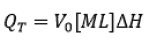(1)

where V0 is the volume of the calorimetric cell, and [M]T is the total concentration of macromolecule in the cell. The first condition implies that the enthalpy of interaction (i.e. enthalpy for complex formation) is different from zero. The second condition is fulfilled from the first condition, as QT is proportional to advance of the binding process: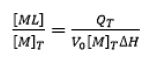(2)

It is possible to use Equations 1 and 2 in a model-free manner for estimation of the binding enthalpy if the following conditions are met:

• Complete saturation of the macromolecule is achieved or ML/MT is close to 1
• Suitable subtraction of the reference background heats is performed
• Protein concentration is determined with reasonable accuracy

In comparison with other biophysical techniques, the key advantages are:

• Complete basic thermodynamic characterization (stoichiometry, association constant, and binding enthalpy) in a single experiment
• Heat is a universal signal, and, hence, no need for reporter labels (e.g. chromophores, fluorophores)
• Direct determination of the binding enthalpy
• Non-destructive technique
• Interaction in solution
• Possibility of performing experiment with optically dense solutions or unusual systems (e.g. dispersions, intact organelles or cells)
• Considerably fast (from 0.25 h/assay in the single injection experiment to 2 h/assay in the conventional titration experiment).

• Signal is proportional to the binding enthalpy, and the non-covalent complexes may exhibit rather small binding enthalpies
• Heat is a universal signal and each process contributes to the global measured heat, thus complicating the evaluation of the contribution because of binding
• Large amount of sample is needed, though this has come down considerably
• It is a slow technique with a low throughput (0.25 - 2 h/assay), not suitable for HTS
• Kinetically slow processes may be overlooked
• A limited range for consistently measured binding affinities (association constants from 104 - 10M-1 for the traditional titration experiment
• Before now, no kinetic information for binding interactions could be accessed

## Basic Equilibrium Thermodynamics

When a macromolecule with a single binding site and a ligand are mixed at certain experimental concentrations, the extent of the reversible binding reaction, or the concentration of the macromolecule-ligand complex, [ML], is given by the equilibrium association constant, Ka: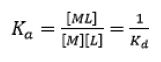(3)

where Kd is the equilibrium dissociation constant for the complex, [M] and [L] are the concentrations of free macromolecule and ligand respectively. Both the constants are associated with the (standard) Gibbs energy of binding, ΔG: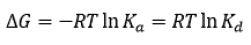(4)

where T is the thermodynamic or absolute temperature and R is the gas constant.

The larger the association constant, the smaller dissociation constant and the more negative the Gibbs energy of binding, resulting in a stronger complex.

Equation 3 results in the well-known hyperbolic relationship between the molar fraction of macromolecule-ligand complex and the concentration of the free ligand: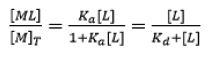(5)

From this equation, it is possible to derive the concentration of macromolecule-ligand complex formed and, hence, the heat associated with that formation could be readily computed just by multiplying by the total protein concentration and the molar binding enthalpy. However, the free ligand concentration is unknown in a titration experiment and the controlled concentrations are the total concentration of macromolecule and ligand. Hence, Equation 5 cannot be used in titration analysis.

The binding Gibbs energy can be partitioned into its enthalpic, ΔH, and entropic, TΔS, contributions: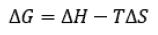(6)

The basic thermodynamic binding profile for a specific interaction includes the three key binding parameters, Gibbs energy (calculated from the equilibrium association constant Ka), enthalpy and entropy of binding. Combining these three thermodynamic potentials offers basic and valuable information on the binding process.

## Direct Measurement of Binding Thermodynamics

As shown before, a single ITC experiment offers the complete basic thermodynamic profile for a given binding interaction. The experimental methodology involves performing several titrant injections (usually the ligand) from a syringe into the titrand solution (usually the macromolecule) in the cell in a completely automated fashion, while maintaining the system at isobaric, quasi-isothermal conditions.

Through sequential injection of the ligand, the chemical equilibrium is disturbed by the incremental changes in the reactant concentrations triggered by the ligand injections, and the system undergoes several equilibrium states that differ in their composition, as the partition between free macromolecule and ligand-bound macromolecule is based on the equilibrium (association or dissociation) constant and the total concentrations of macromolecule and ligand: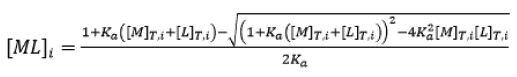(7)

where the subscript i shows the injection number along the titration, and the total concentrations of macromolecule and ligand after each injection are given by: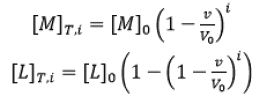(8)

where [M]0 and [L]0 are the initial macromolecule concentration in the cell and the concentration of ligand in the syringe, respectively, v is the injection volume, and the (1- v/V0) factor is the dilution occurring in a calorimetric cell operating at constant volume.

Every transition between two successive states, triggered by ligand injection, is associated with absorption or release of heat proportional to the change in the concentration of complex due to that injection and the molar enthalpy: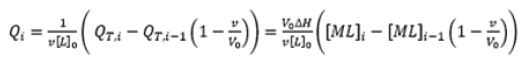(9)

ITC, is a finite-difference or incremental technique.

Figure 1 shows a typical calorimetric titration for a macromolecule with a single ligand binding site.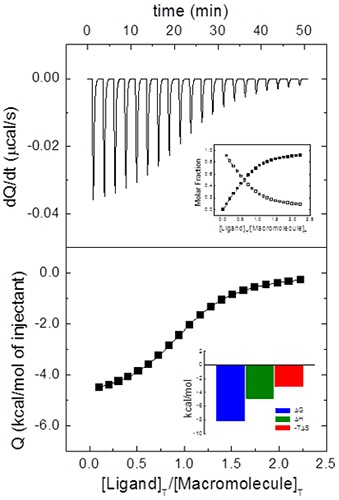Figure 1. Calorimetric titration for a macromolecule binding a ligand with the following binding parameters: Ka = 106 M-1, ΔH = -5 kcal/mol. Upper plot: thermogram (thermal power required to maintain a zero temperature difference between reference and sample cells in the calorimeter) showing the characteristic sequence of peaks corresponding to each ligand injection. Lower plot: binding isotherm (normalized heats per peak as a function of molar ratio, [L] T /[M]T , in the calorimetric cell. Upper inset: evolution of the molar fraction of free macromolecule (open squares) and macromolecule-ligand complex (closed squares) in the calorimetric cell as the titration progresses. Lower inset: thermodynamic binding profile for the macromolecule-ligand interaction; in this case both enthalpic and entropic contributions are favorable to the binding. As it can be clearly observed, the average value of the last peaks is not a good estimate of the injection background heat (the so-called "heat of dilution").

It is possible to normalize the nominal protein concentration using the parameter N in for obtaining a non-fractional N value (Figure 2), hence, "titrating" the protein solution.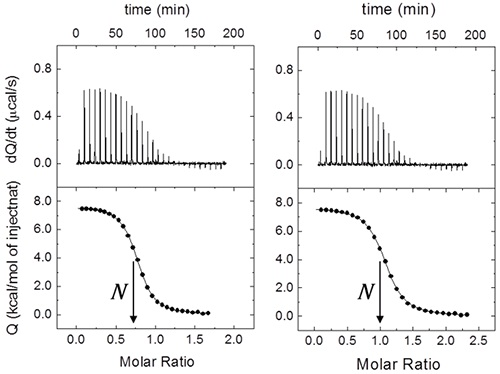Figure 2. Effect of macromolecule concentration uncertainty on the binding parameters estimation from a calorimetric titration. (Left) A fractional value of N (0.72 ± 0.01) is obtained from the data analysis using the nominal concentrations (230 pM for the ligand in the syringe, and 29 pM for the macromolecule in the cell). Because in this case the ligand concentration is considered to be fairly accurate, the fractional value indicates that the protein concentration has been estimated in excess with a 28% error. Employing a protein concentration normalized according to N (21 µM), the expected non- fractional 1:1 stoichiometry (N ≈ 1) is recovered (Right). Because only the cell concentration has been modified, the association constant and the binding enthalpy remain unaltered. If the syringe concentration is modified, both parameters will also change.

For appropriately applying Equation 9 in analyzing an experimental titration, and obtaining good estimates of the binding parameters, the "heat of dilution" problem needs to be addressed.

There are three ways for removing the contribution of this unspecific heat effect:

• Performing a control experiment by the dilution of the ligand into buffer and subtracting the resulting heats from the ligand-macromolecule titration;
• Averaging the heats associated to the last injections (close to macromolecule saturation) in the ligand-macromolecule titration and subtracting that value from the data
• Introducing into Equation 9 an additional adjustable parameter, Qd, accounting for the injection background heat

The third option is the one preferred as the dilution experiment does not necessary mimic the injection background effect when a macromolecule is present.

## Quantitative Information Accessible by ITC

In theory, it is possible to determine the equilibrium association constant, the binding enthalpy and the stoichiometry from a single titration.

However, the consistency of that estimation is based on the binding affinity and the experimental setup. A dimensionless parameter c can be set up, where:

c = Ka[M]0= [M]0/Kd

• If 1 < c < 10000, the three binding parameters can be concurrently and precisely determined
• If c > 10000, only the binding enthalpy and the stoichiometry can be determined
• If c < 1, only the equilibrium association constant can be determined, as the binding enthalpy and the stoichiometry will be correlated

Figure 3 shows the three different scenarios based on the value of c.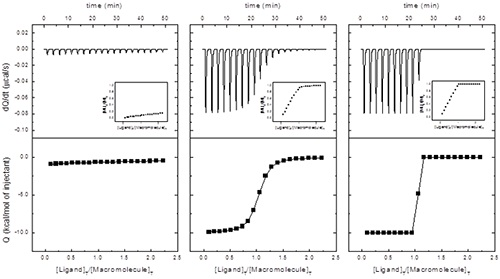Figure 3. Calorimetric titrations at different c values: (left) c = 0.1, Ka = 104 M-1; (middle) c = 100, Ka 107 M- 1; (right) c = 100000, Ka = 1010 M-1. In all three cases: ΔH = -10 kcal/mol, [M]0 = 10 pM, [L]0 = 100 pM, V0 = 0.2 mL, and v = 2 pL. Insets show the molar fraction of the macromolecule-ligand complex (macromolecule saturation) as the titration progresses. It is clear that the case with low c will be problematic: very low signal-to-noise ratio and a featureless binding isotherm with potential correlation between fitting parameters. Also, the case with high c will be problematic: no possibility of discriminating binding affinities and only a lower limiting value for the association constant could be estimated. The values of c and ΔH determine the geometrical features of the binding isotherm. In particular, the global deflection of the binding isotherm (difference between the intercept with the y-axis and the heat observed at high saturation, i.e. background or "dilution" heat) is directly related to binding enthalpy, and it is equal to c/(c+1)ΔH.The slope of the binding isotherm at a molar ratio of 1 is directly related to the binding affinity and the binding enthalpy, and it is roughly equal to -0.25c1/2ΔH. The binding isotherm will have an inflection point if c >1.

As the titration curvature is based on the parameter c, given a binding affinity, it is possible to modulate that curvature by selecting the macromolecule concentration. Even though the ideal situation is 1 < c < 10000, it is not always possible to work within this window, as it may mean using very low reactant concentrations. In case the experimental system shows an increased (c > 10000) or very low (c < 1) affinity, displacement titrations, including a moderate affinity ligand, help overcome the difficulty.

The experimental procedure can be setup as follows:

• Perform two titrations by the injection of each ligand into a macromolecule
• Perform a third titration by injecting one of the ligands into the macromolecule pre-bound to the second ligand (second ligand in excess, if possible)
• All titrations, binary and ternary, can be analyzed with the single binding site model

The binding heat capacity at constant pressure is the temperature derivative of the binding enthalpy: neither independently nor competitively. Figure 4 shows this procedure in two experimental systems.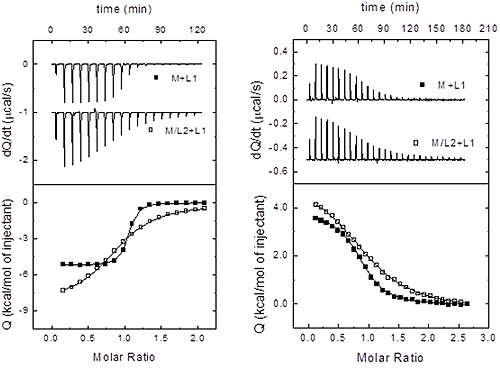Figure 4. Assessing heterotropic interactions by ITC. For a macromolecule binding two different ligands, three scenarios are possible: independent binding, cooperative binding and competitive binding (maximal negative cooperativity)(Left) Titration of macromolecule (30 pM) with ligand 1 (480 pM) in the absence (closed squares) and the presence (220 pM, open squares) of ligand 2. The analysis of both titrations using a model with a single binding site for ligand 1 provides the following parameters: Ka1 = 1.1-107 M-1, ΔH1 = -5.2 kcal/mol, Ka12 = 3.1-101 M-1, ΔH12 = -8.4 kcal/mol. A third titration with ligand 2 (not shown) provides the following parameters: Ka2 = 1.5-105 M-1, ΔH2 = 3.1 kcal/mol. Because Eq. 15 does not hold, the binding of ligand 1 and ligand 2 does not occur independently. On the other hand, considering 205 pM as the average concentration for free ligand2 along the titration with ligand 1, applying Eq. 16 values for the binding parameters for ligand 1 in the presence of ligand 2 can be calculated, Ka12 = 3.5-105 M-1 and ΔH12 = -8.2 kcal/mol, which are very close (within experimental error) to those directly determined (that is, Eq. 16 is fulfilled). Therefore, it can be concluded that ligand 1 and ligand 2 bind competitively (a ≈ 0, Ah ≈ 0). (Right) Titration of macromolecule (21 pM) with ligand 1 (260 pM) in the absence (closed squares) and the presence (50 pM, open squares) of ligand 2. The analysis of both titrations using a model with a single binding site for ligand 1 provides the following parameters: Ka1 = 9.2-105 M-1, ΔH1 = 3.7 kcal/mol, Ka12 = 2.3-105 M-1 , ΔH12 = 5.3 kcal/mol. A third titration with ligand 2 (not shown) provides the following parameters: Ka,2 = 2.7•105 M-1, ΔH2 = -2.1 kcal/mol. Because Eq. 15 does not hold, the binding of ligand 1 and ligand 2 does not occur independently. On the other hand, considering 40 µM as the average concentration for free ligand 2 along the titration with ligand 1, applying Eq. 16 values for the binding parameters for ligand 1 in the presence of ligand 2 can be calculated, Ka,12 = 7.8•104 M-1 and ΔH12 = 5.6 kcal/mol, which are significantly different (within experimental error) to those directly determined (that is, Eq. 16 is not fulfilled). Therefore, it can be concluded that ligand 1 and ligand 2 bind cooperatively. Because the binding affinity of ligand 1 decreases by the presence of ligand 2, the binding cooperativity is negative. Using Eq. 16 the cooperativity parameters can be estimated: α = 0.18, Δh = 1.6 kcal/mol (i.e. if ligand 2 is bound to the macromolecule, the binding affinity of ligand 1 is reduced by a factor of 5, and its binding enthalpy is increased by 1.6 kcal/mol).

By performing ITC experiments at the same conditions such as pH, temperature, ionic strength, and using buffers with different ionization enthalpies, it is possible to assess proton exchange processes coupled to ligand binding, and estimate the buffer-independent binding enthalpy and the net number of protons exchanged. Figure 5 shows this experimental procedure.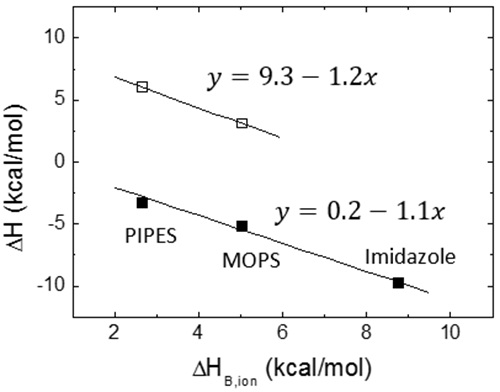Figure 5. Proton exchange coupled to ligand binding constitutes the molecular basis for the pH dependency of ligand binding interactions. Calorimetric titrations were performed for two different ligands (open and close squares) under the same conditions (25°C, pH 7, NaCl 150 mM) employing buffers with similar pKa but different ionization enthalpies (PIPES 2.68 kcal/mol, MOPS 5.05 kcal/mol, imidazole 8.77 kcal/mol). Because the measured enthalpy is markedly dependent on the ionization enthalpy of the buffer with a negative slope, it is concluded that the formation of the macromolecule-ligand complex is accompanied by a net deprotonation. The slope is similar for both ligands, close to 1, and, therefore, at least one proton is released from the complex to the bulk solution. However, the buffer-independent biding enthalpy is significantly different for both ligands: 9.3 kcal/mol and 0.2 kcal/mol. In the vicinity of pH 7 (at least | ApH | < 2), according to Eq. 13 an increase/decrease in pH will lead to an increase/decrease in the binding affinity for both ligands; in particular, a change of 1 unit in pH will increase/decrease the equilibrium association constant by a factor of 10, approximately.

Figure 6 shows the temperature dependency of the thermodynamic binding profile for a specific binding interaction, assuming a constant binding heat capacity.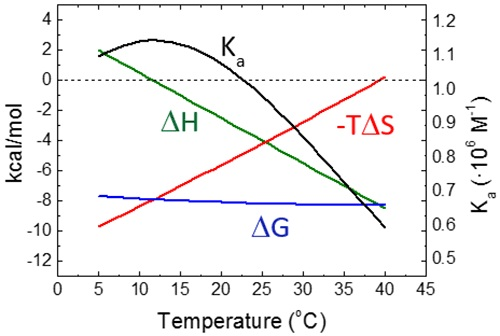Figure 6. Temperature dependency of the thermodynamic binding profile for a macromolecule-ligand interaction with the following binding parameters: Ka = 106 M-1, AG = -8.2 kcal/mol, ΔH = -4 kcal/mol, -TAS = -4.2 kcal/mol, and ACP = -0.3 kcal/mol. The slope of ΔH is equal to ACP, while the slope of -TAS is -(ACP+AS); because usually ACP is considerably larger than AS (within the experimental temperature range), the slopes of the enthalpic and the entropic contributions are very similar, resulting in a Gibbs energy of binding rather insensitive to temperature. Accordingly, it can be observed that the equilibrium association constant shows very little variation with temperature, which, together with the usual experimental uncertainty, makes the estimation of the binding enthalpy through the van't Hoff relationship very challenging. The binding enthalpy is zero at temperature TH (in this case, close to 12°C), which is the temperature for maximal equilibrium association constant, according to the van't Hoff relationship. The entropic contribution is zero at temperature TS (in this case, close to 39°C), which is the temperature for maximal Gibbs energy of binding.

## Conclusion

ITC is a powerful tool for characterizing interactions, especially interactions of biomolecules in a broad range of binding affinities. The different fields and applications for ITC are:

• Protein regulation and function
• Protein engineering and function redesign
• Characterization of pharmacological/biotechnological targets
• Lead selection in drug discovery
• Lead optimization in drug development
• Development of drug carriers for enhanced therapeutic administration
• Pre-clinical assays (e.g. plasma protein binding, potential side-effects through unwanted targets)
• Experimental benchmark for development and refinement of computational models for protein interactions
• Quality control for protein/biologics production in pharmaceutical, cosmetic, food, cleaning and biotech industry.This information has been sourced, reviewed and adapted from materials provided by Malvern Panalytical.

## Citations

• APA

Malvern Panalytical. (2019, September 03). The Working Principle of Isothermal Titration Calorimetry. AZoM. Retrieved on October 29, 2020 from https://www.azom.com/article.aspx?ArticleID=12258.

• MLA

Malvern Panalytical. "The Working Principle of Isothermal Titration Calorimetry". AZoM. 29 October 2020. <https://www.azom.com/article.aspx?ArticleID=12258>.

• Chicago

Malvern Panalytical. "The Working Principle of Isothermal Titration Calorimetry". AZoM. https://www.azom.com/article.aspx?ArticleID=12258. (accessed October 29, 2020).

• Harvard

Malvern Panalytical. 2019. The Working Principle of Isothermal Titration Calorimetry. AZoM, viewed 29 October 2020, https://www.azom.com/article.aspx?ArticleID=12258.